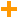Block Group: Math Operations Icon:For information on using dataflow blocks, see Dataflow.

For accepted and excluded arguments of Math Operations blocks, see Math Operations.

## Input/Output Property

The following property of the Add block can take input and give output.

• input n (number)

input n specifies one of the numbers to add.

## Output Property

The following property of the Add block can give output but cannot take input.

• output (number)

output returns the result of adding all of the input values.

## Examples

The following image shows two examples of the Add block. In the leftmost Add block, two number values are added. In the rightmost Add block, three different data types are added: a number, a string representation of a number, and a Boolean value.